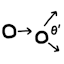#Angles in elastic two-body collisions

In high school physics we learned about momentum, kinetic energy, and elastic collisions. Here is a remarkable fact: Suppose we have two objects with the same mass. Object one is stationary, whereas object two is moving toward object one. After they collide and assuming the collision is perfectly elastic, the two objects will always depart at a right angle to each other.

We can prove this fact by applying the conservation of momentum (physical law), the conservation of energy (true iff the collision is perfectly elastic), and the law of cosines (pure math). Furthermore we can show that if the two objects have unequal mass, then they must depart at a non-right angle.

## Givens

Variables:

• $$\vec{v}_{1i}$$: Initial velocity vector of first object, non-zero.
• $$\vec{v}_{1f}$$: Final velocity vector of first object.
• $$m_1$$: Mass of first object, greater than zero.
• $$\vec{v}_{2i} = \vec{0}$$: Initial velocity vector of first object, must be zero.
• $$\vec{v}_{2f}$$: Final velocity vector of second object.
• $$m_2$$: Mass of second object, greater than zero.

Laws to use:

• $$m_1 \vec{v}_{1i} + m_2 \vec{v}_{2i} = m_1 \vec{v}_{1f} + m_2 \vec{v}_{2f}$$: Conservation of momentum.
• $$\frac{1}{2} m_1 |\vec{v}_{1i}|^2 + \frac{1}{2} m_2 |\vec{v}_{2i}|^2 = \frac{1}{2} m_1 |\vec{v}_{1f}|^2 + \frac{1}{2} m_2 |\vec{v}_{2f}|^2$$: Conservation of energy.
• $$c^2 = a^2 + b^2 - 2 a b \cos \theta$$: Law of cosines.

## Proof

From the conservation of momentum, the three vectors $$c = \vec{v}_{1i}$$, $$a = \vec{v}_{2i}$$, and $$b = k \, \vec{v}_{2f}$$ form the sides of a triangle, defining $$k = \frac{m_2}{m_1} > 0$$:

$$m_1 \vec{v}_{1i} + m_2 \cdot \vec{0} = m_1 \vec{v}_{1f} + m_2 \vec{v}_{2f}$$. (Substitute $$\vec{v}_{2i}$$)
$$m_1 \vec{v}_{1i} = m_1 \vec{v}_{1f} + m_2 \vec{v}_{2f}$$. (Simplify)
$$\vec{v}_{1i} = \vec{v}_{1f} + \frac{m_2}{m_1} \vec{v}_{2f}$$. (Divide by $$m_1$$)
$$\vec{v}_{1i} = \vec{v}_{1f} + k \vec{v}_{2f}$$. (Substitute $$k$$)

Apply the conservation of energy:

$$\frac{1}{2} m_1 |\vec{v}_{1i}|^2 + \frac{1}{2} m_2 |\vec{v}_{2i}|^2 = \frac{1}{2} m_1 |\vec{v}_{1f}|^2 + \frac{1}{2} m_2 |\vec{v}_{2f}|^2$$.
$$m_1 |\vec{v}_{1i}|^2 + m_2 |\vec{v}_{2i}|^2 = m_1 |\vec{v}_{1f}|^2 + m_2 |\vec{v}_{2f}|^2$$. (Multiply by 2)
$$m_1 |\vec{v}_{1i}|^2 + m_2 |\vec{0}|^2 = m_1 |\vec{v}_{1f}|^2 + m_2 |\vec{v}_{2f}|^2$$. (Substitute $$\vec{v}_{2i}$$)
$$m_1 |\vec{v}_{1i}|^2 = m_1 |\vec{v}_{1f}|^2 + m_2 |\vec{v}_{2f}|^2$$. (Simplify)
$$|\vec{v}_{1i}|^2 = |\vec{v}_{1f}|^2 + \frac{m_2}{m_1} |\vec{v}_{2f}|^2$$. (Divide by $$m_1$$)
$$|\vec{v}_{1i}|^2 = |\vec{v}_{1f}|^2 + k |\vec{v}_{2f}|^2$$. (Substitute $$k$$)

Apply the law of cosines:

$$|\vec{v}_{1i}|^2 = |\vec{v}_{1f}|^2 + |k \vec{v}_{2f}|^2 - 2 |\vec{v}_{1f}| |k \vec{v}_{2f}| \cos \theta$$. (Substitute $$a$$, $$b$$, $$c$$)

Now subtract the previous two equations:

$$\require{cancel} \cancel{|\vec{v}_{1i}|^2} - \cancel{|\vec{v}_{1i}|^2} = (\cancel{|\vec{v}_{1f}|^2} + |k \vec{v}_{2f}|^2 - 2 |\vec{v}_{1f}| |k \vec{v}_{2f}| \cos \theta) - (\cancel{|\vec{v}_{1f}|^2} + k |\vec{v}_{2f}|^2)$$.
$$0 = |k \vec{v}_{2f}|^2 - 2 |\vec{v}_{1f}| |k \vec{v}_{2f}| \cos \theta - k |\vec{v}_{2f}|^2$$. (Cancel)
$$2 |\vec{v}_{1f}| |k \vec{v}_{2f}| \cos \theta = |k \vec{v}_{2f}|^2 - k |\vec{v}_{2f}|^2$$. (Rearrange)
$$2 k |\vec{v}_{1f}| |\vec{v}_{2f}| \cos \theta = k^2 |\vec{v}_{2f}|^2 - k |\vec{v}_{2f}|^2$$. (Simplify)
$$2 |\vec{v}_{1f}| \cos \theta = k |\vec{v}_{2f}| - |\vec{v}_{2f}|$$. (Divide by $$k |\vec{v}_{2f}|$$)
$$2 |\vec{v}_{1f}| \cos \theta = |\vec{v}_{2f}| (k - 1)$$. (Factor)
$$\cos \theta = \frac{|\vec{v}_{2f}| (k - 1)}{2 |\vec{v}_{1f}|}$$. (Divide)
$$\theta = \cos^{-1} \frac{|\vec{v}_{2f}| (k - 1)}{2 |\vec{v}_{1f}|}$$. (Inverse cosine)

We know that $$\cos^{-1} x = 90^\circ$$ if and only if $$x = 0$$. For this to happen, one or more terms in the numerator must be zero, i.e. $$|\vec{v}_{2f}| = 0$$ or $$k - 1 = 0$$. The first case is uninteresting because it implies no collision happened, and the division operation was invalid anyway. So the second case is logically equivalent to $$k = 1$$, equivalent to $$m_2 / m_1 = 1$$, thus equivalent to $$m_2 = m_1$$. Therefore due to the logical equivalence, when $$m_2 \neq m_1$$ we have $$x \neq 0$$, hence $$\cos^{-1} x \neq 90^\circ$$.

## Notes

Note that $$\theta$$ is the angle between vectors $$\vec{v}_{1f}$$ and $$\vec{v}_{2f}$$ when they are placed head-to-tail. The angle between vectors when placed tail-to-tail is the supplementary angle of $$180^\circ - \theta$$. But we know that $$\theta = 90^\circ$$ if and only if $$180^\circ - \theta = 90^\circ$$, so the argument still holds.

Also note that the right angle property does not apply in the degenerate cases when no collision happens ($$\vec{v}_{1f} = \vec{v}_{1i}$$ and $$\vec{v}_{2f} = \vec{0}$$), or when the first object comes to complete stop and the second object picks up the full velocity ($$\vec{v}_{1f} = \vec{0}$$ and $$\vec{v}_{2f} = \vec{v}_{1i}$$).